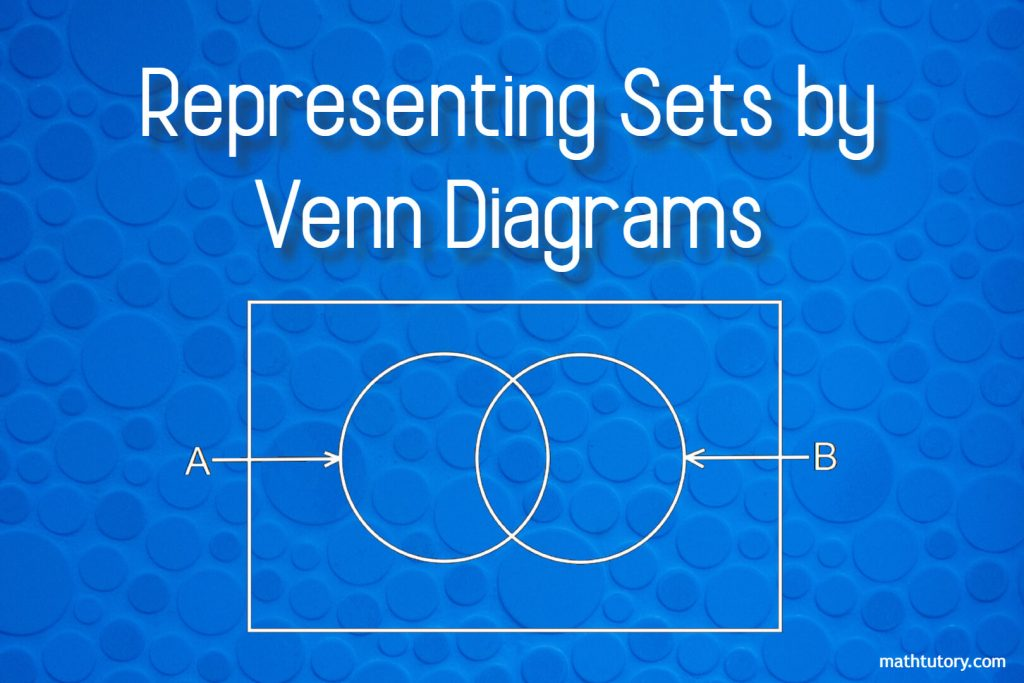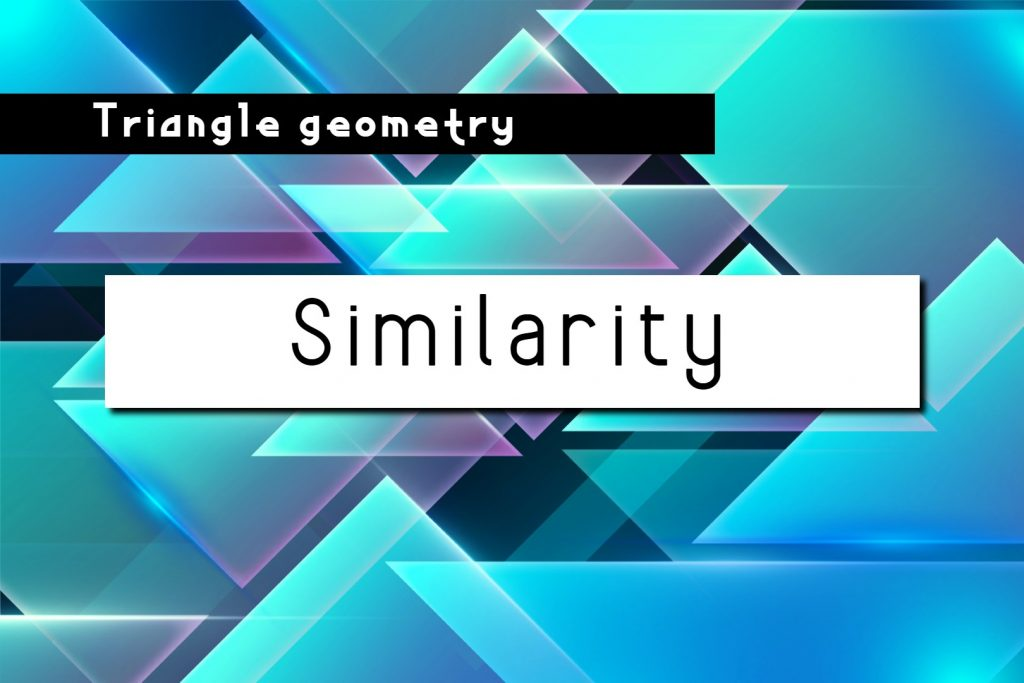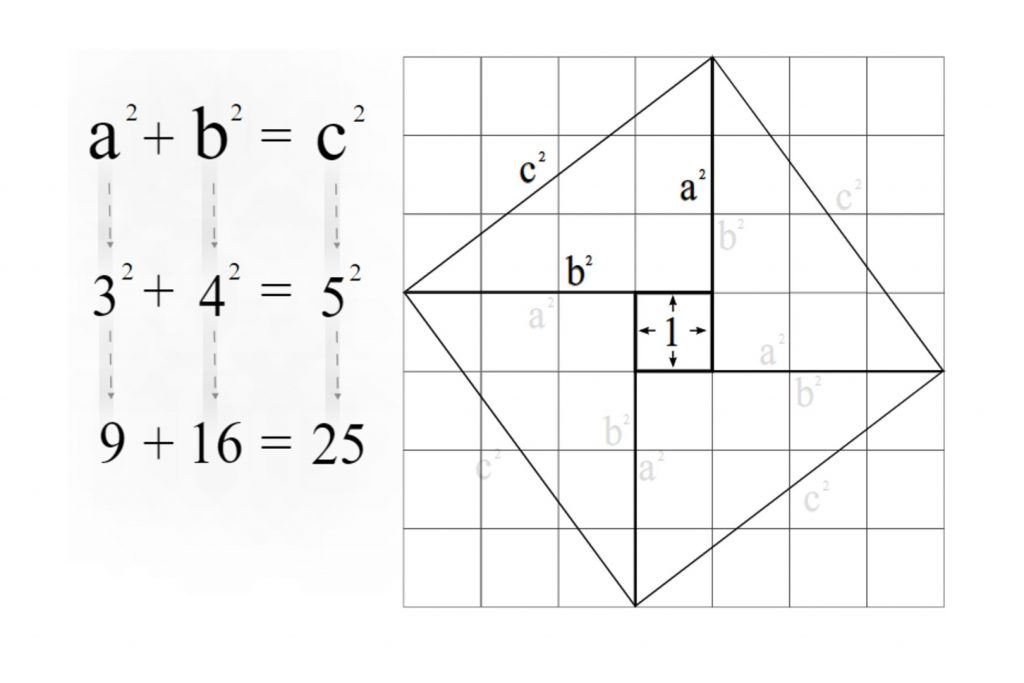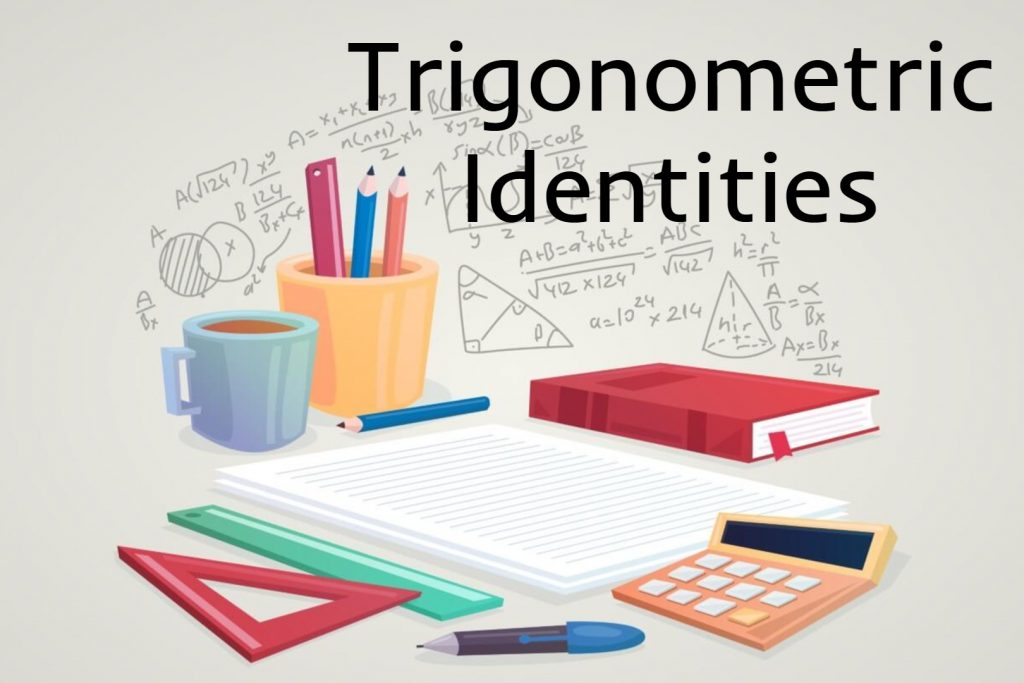## Grade 7 – Unit 08 – Directed Numbers

Do the following exercises using number line. Exercise 8.1 in page number 83 Exercise 8.2 in page number 84 Exercise 8.3 in page number 85

## Grade 6 – Unit 06 – Rounding Off

https://youtu.be/z0wy5fs4Vm8 Write this note in your exercise book. Rounding Off When rounding off a number to the nearest multiple of ten, if the digit in the ones place of the number is less than 5 (1,2,3,4), then the closest multiple of ten which is less than that number is selected. is 5 or greater than …## Representing Sets by Venn Diagrams

Venn diagrams are the most common way of representing a set. This method was introduced by the English mathematician John Venn. Therefore these are called Venn Diagram. Set is a collection of items that can be clearly identified.The items in a set are called its elements. Example: Let A be the set of even numbers …## Similarity

Similarity,In what follows, we’ll see that many—if not most—of our results shall rely on the proportionality of sides in similar triangles. A convenient statement is as follows. Similarity, Given the similar triangles ΔABC ∼ ΔA’BC’,We have that, Conversely, if then triangles ΔABC ∼ ΔA’BC’ are similar. Proof Note first that ΔAA’C’ and ΔCA’C’ clearly have …## The Pythagorean Theorem

Right-angled triangle and the Pythagorean theorem In this article lets try to develop the Pythagorean theorem by means of the right angle. So first let’s get some proper ideas about the right-angled triangle. What is a right-angled triangle? If an angle of a triangle is 90o it is called a right-angled triangle or right triangle. …## Trigonometric Identities

A Trigonometric identity or trig identity is an identity that contains the trigonometric functions sine(sin), cosine(cos), tangent(tan), cotangent(cot), secant(sec), or cosecant(csc). Trigonometric identities can use to: Simplify trigonometric expressions. Solve trigonometric equations. Prove that one trigonometric expression is equivalent to another so that we can replace the first expression by the second expression. The following …